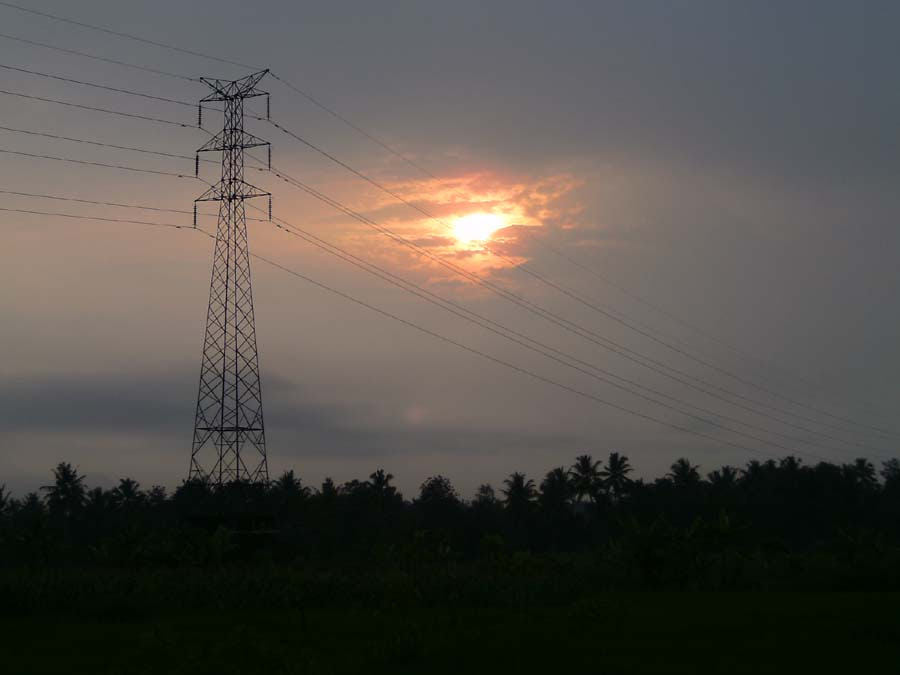# High Voltage Electrical Transmission

Electrical energy generated, generally produces a voltage of 10 kV. Before distributing electric power, a trafo or transformers in generating stations will raise the voltage to 66 kV, 132 kV, or even 400 kV. Electric power in Indonesia are distributed through the grid at this voltage level.Before delivery to the customer, local transformer will reduce the voltage (gradually) to 220 V. The final phase of the voltage transformer will be 220 ​​V for use by a group of users, can be performed by a transformer mounted on the poles.

The transformer plays a vital role in the process of distribution of power. Transformer can only work with AC power. This is one reason why the electricity supplied in the form of alternating current.

Power losses
The power loss that occurs during the transmission process can be calculated as follows. Suppose that the resistance power cord that connects the station to a house is 1 Ω. Suppose that a variety of electrical devices in that house, using a 6 kW power rating. Assume that the current is distributed with source voltage of 220 V.

First calculate the electric currents
I = P / V = 6 kW / 220 V = 6000 W / 220 V = 27.3 A

Then calculate the power loss that occurs on the cable
P = I2 x R = (27.32 A)2 x 1 Ω = 745.29 W

Percentage power loss of all power supplied to the house, becomes
745.29 W / 6000 W x 100% = 12%

Now, consider what happens when the voltage is raised to 132 kV.

At higher voltages, the magnitude of electric current is
I = P / V = 6 kW / 132 kV = 6000 W / 132000 V = 0.045 A

The power loss that occurs on the cable
P = I2 x R = (0.045 A)2 x 1 Ω = 0.002 W

Percentage power loss of all power supplied to the house, becomes
0.002 W / 6000 W x 100% = 0.00003%

The amount of power loss 132 kV transmission voltage is 0.00003% of the total power delivered to the house. So that we can ignore the losses that occur in a relatively short distance after the voltage is lowered to 220 V. This is the main reason why electric energy distributed at high voltage.

Next→
←Prev

### Choose label

1.2.3.